AP State Syllabus AP Board 8th Class Maths Solutions Chapter 3 Construction of Quadrilaterals Ex 3.1 Textbook Questions and Answers.

## AP State Syllabus 8th Class Maths Solutions 3rd Lesson Construction of Quadrilaterals Exercise 3.1Construct the quadrilaterals with the measurements given below:

Question (a).
Quadrilateral ABCD with AB = 5.5 cm, BC = 3.5 cm, CD = 4 cm, AD = 5 cm and ∠A = 45°.
Solution:
In Quadrilateral ABCD with AB = 5.5 cm, BC = 3.5 cm, CD = 4 cm, AD = 5 cm and ∠A = 45°.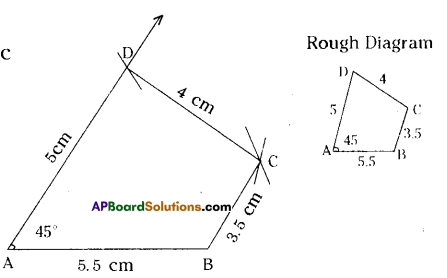Construction Steps:

1. Construct a line segment $$\overline{\mathrm{AB}}$$ with radius 5.5 cm
2. With the centre A draw a ray and an arc which are equL1 to 45° and 5 cm.
3. These intersecting point is keep as ‘D’.
4. With centres D, B draw two arcs equal to radius 4 cm, 3.5 cm respectively.
5. The intersecting point of these two arcs is keep as ‘C’.
6. Join DC and BC. A F
7. ∴ The required quadrilateral ABCD is formed.

Question (b).
Quadrilateral BEST with BE = 2.9 cm, ES = 3.2 cm, ST = 2.7 cm, BT = 3.4 cm and ∠B=75°.
Solution:
BE = 2.9 cm, ES = 3.2 cm, ST = 2.7 cm, BT = 3.4 cm and ∠B=75°.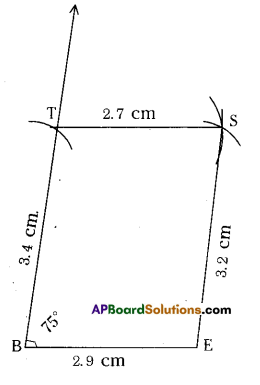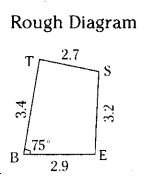Construction Steps:

1. Draw a line segment $$\overline{\mathrm{BE}}$$ with radius 2.9 cm.
2. With the centre B, draw a ray of 75° and draw 2.9
an arc with radius 3.4 cm, keep the intersecting point of these two as T.
3. With the centres T, E draw arcs with radius 2.7 cm, 3.2 cm respectively. These intersection point is keep as S’.
4. Join T, S and E,S.
5. ∴ The required quadrilateral BEST is formed.

Question (c).
Parallelogram PQRS with PQ = 4.5 cm, QR =3 cm and ∠PQR = 60°.
Solution: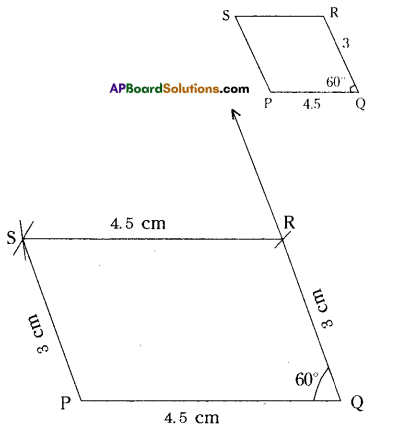In a parallelogram PQRS
PQ = 4.5 cm, QR = 3 cm, ZPQR = 60°.
=RS4.5cmzPS=3crn
[: Opposite sides of a I)aralielograrn are equal]

Construction Steps:

1. Draw a line segment ¡i with radius 4.5 cm.
2. With the centre Q draw a ray and an arc equal to 60° and 3 cm.
3. The intersecting point of these two keep as R’.
4. With the centres R, P draw arcs with 4.5 cm, 3 cm respectively. Keep ‘S’ as the intersecting point of these two arcs.
5. Join P, S and R, S.
6. ∴ The required parallelogram PQRS is formed.

Question (d).
Rhombus MATH with AT =4 cm, ∠MAT =120°.
Solution: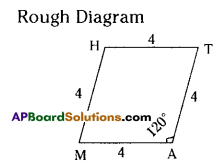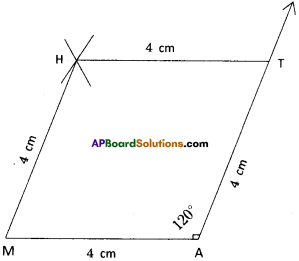Construction Steps:

1. Draw a line segment $$\overline{\mathrm{MA}}$$ with radius 4 cm.
2. With the centre A draw a ray and an arc equal to 120°, 4 cm. These two intersecting point be keep as T.
3. With the centres M, T draw arcs equal to 4 cms.
These two arcs intersected at the point ‘H’.
4. Join M, H and T, H.
5. ∴ The required rhombus MATH is formed.

Question (e).
Rectangle FLAT with FL =5 cm, LA= 3 cm.
Solution: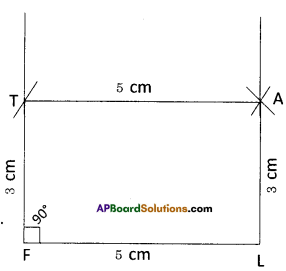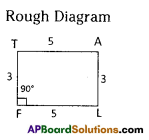In a rectangle FLAT
FL=AT=5cm, LA = TF = 3cm, ∠F = ∠L = ∠A = ∠T = 90°

Construction Steps:

1. Draw a line segment $$\overline{\mathrm{FL}}$$ with radius 5 cm.
2. With the centre F draw a ray and an arc equal to 900, 3 cm.
These to meet at point T.
3. With the centres T, L draw arcs equal to 5 cm, 3 cm respectively.
4. These two arcs meet at the point ‘A’.
5. Join T, A and L, A.
6. ∴ The required rectangle FLAT is formed.

Question (f).
Square LUDO with LU = 4.5 cm.
Solution: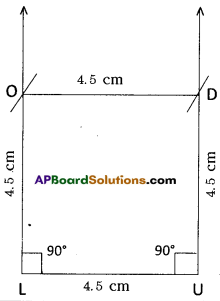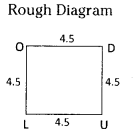In a square LUDO
LU = UD = DO = OL = 4.5 cm
∠L = ∠U = ∠D = ∠O = 90°

Construction Steps: 45

1. Draw a line segment $$\overline{\mathrm{LU}}$$ with radius 4.5 cm.
2. With the centre ‘L’, draw a ray of 90° and an arc with radius 4.5 cm. These two meet at the point ‘O’.
3. Now with the centre U’, draw another ray of 90° and an arc with radius 4.5 cm. These two meet at the point “D”.
4. Join O, D.
5. ∴ The required square LUDO is formed.